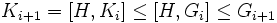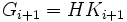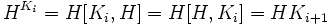# Equivalence of definitions of subnormal subgroup

This article gives a proof/explanation of the equivalence of multiple definitions for the term subnormal subgroup
View a complete list of pages giving proofs of equivalence of definitions

## The definitions that we have to prove as equivalent

1. There exists an ascending chain$H = H_0 \le H_1 \dots H_n = G$ such that each$H_i$ is normal in$H_{i+1}$. The smallest possible$n$ for which such a chain exists is termed the subnormal depth of$H$.
2. Consider the descending chain$G_i$ defined as follows:$G_0 = G$ and$G_{i+1}$ is the normal closure of$H$ in$G_i$. Then, there exists an$n$ for which$G_n = H$. The smallest such$n$ is termed the subnormal depth of$H$.
3. Consider the sequence$K_i$ of subgroups of$G$ defined as follows:$K_0 = G$, and$K_{i+1} = [H,K_i]$ (the commutator), This sequence of subgroups eventually enters inside$H$. The number of steps taken is termed the subnormal depth of$H$.

## Facts used

1. Subnormal subgroup has a unique fastest descending subnormal series
2. Product with commutator equals join with conjugate: If$H \le G$ and$A$ is a subset of$G$, we have$\langle H, H^A \rangle = H[A,H]$.

## Proof

Fact (1) covers the equivalence of definitions (1) and (2). We thus need to prove the equivalence of definitions (2) and (3).

### (2) implies (3)

Given: A subgroup$H$ of$G$. A descending subnormal series for$H$ in$G$ given by$G_0 = G$, and$G_{i+1}$ is the normal closure of$H$ in$G_i$. We have$G_n = H$.

To prove: Consider the sequence$K_0 = G$,$K_i = [H,G_i]$. Then,$K_n \le H$.

Proof: Observe that since$G_{i+1}$ is normal in$G_i$, we have:$[G_{i+1}, G_i] \le G_{i+1}$.

Further, since$H \le G_{i+1}$, we get:$[H,G_i] \le G_{i+1}$.

We now prove, by induction on$i$, that$K_i \le G_i$.

• The base case for induction is true, since for$i = 0$,$K_0 = G_0 = G$.
• The induction step: Suppose$K_i \le G_i$. Then$K_{i+1} = [H,K_i] \le [H,G_i] \le G_{i+1}$.

Thus, if$G_n = H$, we get$K_n \le H$.

### (3) implies (2)

Given: A subgroup$H$ of$G$. A descending chain of subgroups given by$K_0 = G$, and$K_{i+1} = [H,K_i]$. We have$K_n \le H$.

To prove: Define$G_0 = G$, and$G_{i+1}$ as the normal closure of$H$ in$G_i$. Then,$G_n = H$.

Proof: We do this by establishing a relation between$K_i$ and$G_i$ inductively, namely:$G_i = HK_i$.

We prove this inductively. The base case is direct since both sides are equal to$G$ for$i = 0$. We thus need to prove the induction step, i.e., if$G_i = HK_i$, we need to prove that$G_{i+1} = HK_{i+1}$.

By fact (2), we have:$H^{K_i} = H[K_i, H] = H[H,K_i] = HK_{i+1}$.

On the other hand, we have by induction that$G_i = HK_i$, so:$G_{i+1} = H^{G_i} = H^{HK_i} = H^{K_i}$.

Putting these together, we get:$G_{i+1} = HK_{i+1}$,

as desired.

Now that we have the general relation$G_i = HK_i$, it is clear that if$K_n \le H$, we have$G_n = H$.